# Cardano formula

(diff) ← Older revision | Latest revision (diff) | Newer revision → (diff)

A formula for finding the roots of the general cubic equation over the field of complex numbers(1)

Any cubic equation can be reduced to the above form. The Cardano formula for the roots of (1) has the form: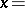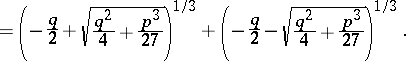In this formula one must choose, for each of the three values of the cube rootthat value of the cube root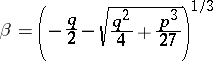for which the relation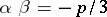holds (such a value ofalways exists). In the Cardano formula,andare arbitrary complex numbers. In the case of real coefficientsand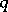, the property of the roots being real or imaginary depends on the sign of the discriminant of the equation,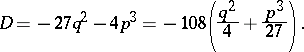Whenall three roots are real and distinct. However, according to Cardano's formula, the roots are expressed in terms of cube roots of imaginary quantities. Although in this case both the coefficients and the roots are real, the roots cannot be expressed in terms of the coefficients by means of radicals of real numbers; for this reason, the above case is called irreducible. When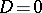, all roots are real; whenandare both non-zero, there is one double and one single root; and whenandare both zero, there is one triple root. When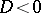, all three roots are distinct, one of them being a real number and the other two — conjugate complex numbers.

The Cardano formula is named after G. Cardano, who was the first to publish it in 1545.

How to Cite This Entry:
Cardano formula. Encyclopedia of Mathematics. URL: http://encyclopediaofmath.org/index.php?title=Cardano_formula&oldid=14215
This article was adapted from an original article by I.V. Proskuryakov (originator), which appeared in Encyclopedia of Mathematics - ISBN 1402006098. See original article